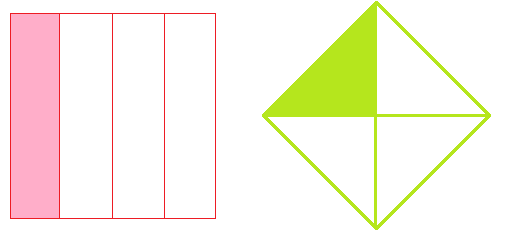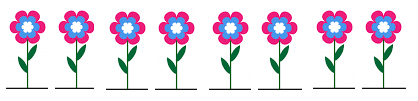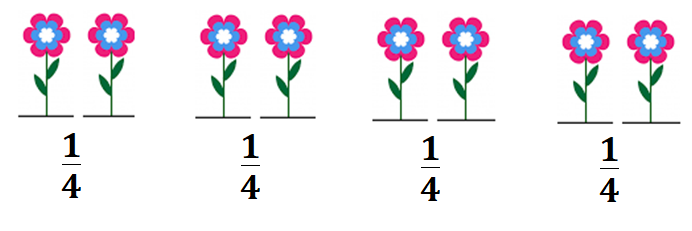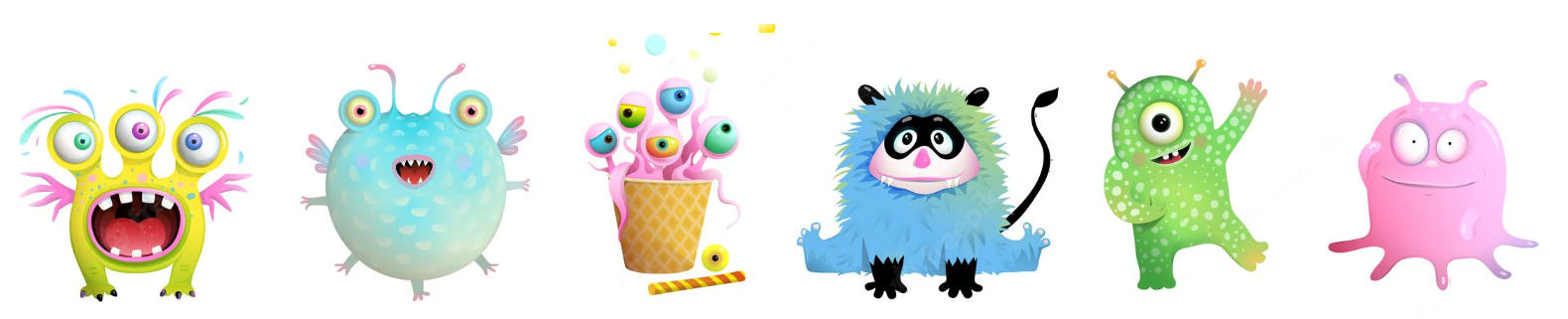# Math > Year 2

## Recognizing ,  and

Quarter
One quarter means one of four equal parts. Four quarters make one whole.

Example:Here, out of 4 equal parts, one part is shaded.
.

To find one quarter, we split the whole into 4 equal parts.

Here we have 8 flowers.To find a quarter of 8 flowers, we will split the 8 flowers into four equal groups.By splitting the 8 flowers into four equal parts, we get 2 flowers in each group.
A quarter means one of four equal parts.

of 8 = × 8 = 2

2/4 of 8 =
+  = 2 + 2 = 4

¾ of 8 =
+ + = 2 + 2 + 2 = 6

To find a quarter of an amount, we divide the amount by 4.

For example:

of 12 = 12 ÷ 4 = 3

Let's try a question.

Here is a quarter of the group of monsters. What is the total number of monsters in the group?Number of above-given monsters = 6

It is a quarter of the group of monsters.

We will add 6 four times to know the whole number.

Four quarters make one whole.

6 + 6 + 6 + 6

= 24

So, the whole is 24.

//